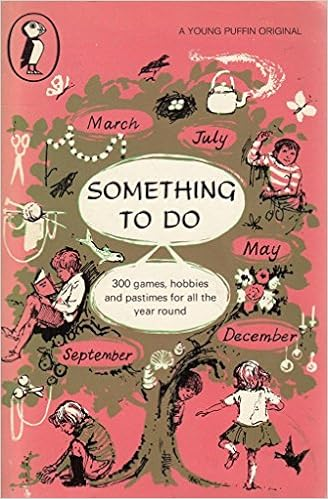Time Travel Post

# Time Travel: An Old Book

Many moons ago I was given a book by an aged Aunt, it was titled ‘Something To Do’. In it had lots of ideas. The pink cover put me off because it was a girls colour but when Mum flicked through it and found how to make a hill for you model railway. Many years on a trip somewhere Mum had squirreled away the book and on a train trip showed me the following article:

Things to do in the Train.

Check The Speed
This only needs a watch with a second hand and a little arithmetical division. If you look out of a train window you will see white posts about a meter high with black numbers set at regular intervals besides the ‘downside’ track (though the posts and markings vary slightly from region to region).
The posts are actually at 1/4 mile intervals and if you note the number of seconds it takes to travel from one signpost to the next you know how long it takes the train to travel a quarter of a mile., By dividing this number of seconds in to 900 you can then work out the speed of your train in miles per hour.

i.e. Let x be the number of seconds it takes the train to to cover the distance between two consecutive posts.
x seconds for 1/4 mile = 4x seconds for 1 mile.
There are 3600 seconds in one hour.
Therefore miles covered in one hour (i.e. speed of train)

 = 3600 = 900 m.p.h. 4x x

If, for example, you find the train takes 9 seconds between two 1/4 mile posts, the sum is then 900 ÷ 9 = 100 m.p.h.
To convert m.p.h. to kilometers use the following table:

 m.p.h. 40 50 60 70 80 90 km.p.h. 65 80 97 113 129 145